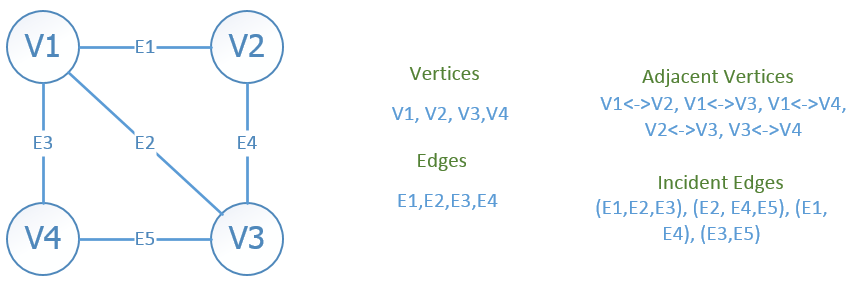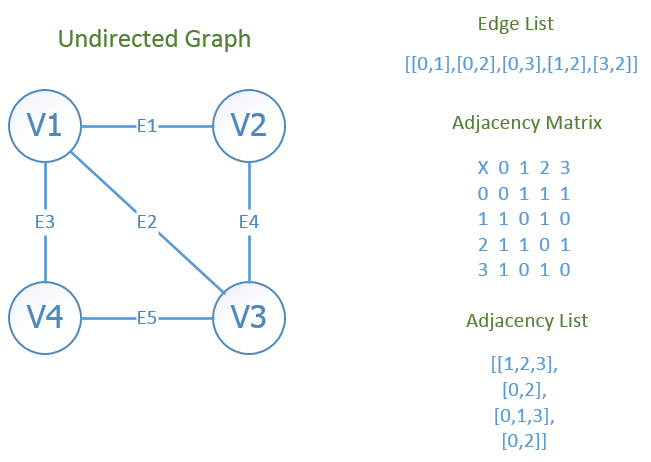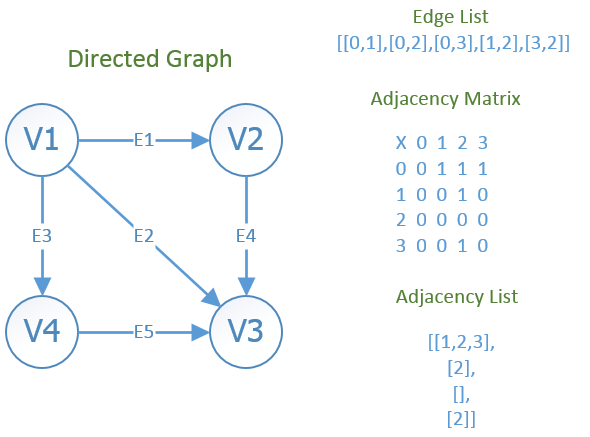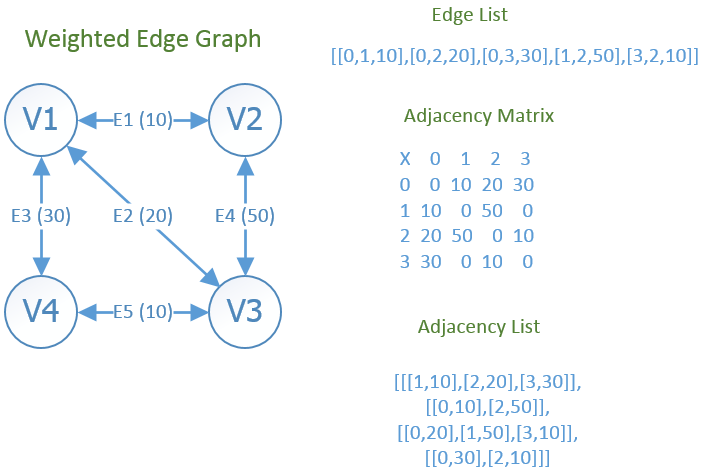Developer

## Graph Data Structure Cheat Sheet

A graph is an abstract data type that consists of a finite set of vertices together with a set of edges connecting the vertices.

## terms

• Vertex/vertices - a point / node in the graph
• Edge - a connection between two vertices
• Adjacent (coincident) - relationship between two vertices. A vertex A is adjacent to vertex B if an edge connects them; Vertex A and B are both incident on edge E
• Incident - a relationship between an edge and a vertex. A vertex and an edge are incident if the vertex is one of the two vertices the edge connects. Also, two or more edges can be called incident if they share a common vertex## Basic Graph Operations

• `adjacent(G,x,y)` - check if edge between x and y
• `neighbors(G,x)` - list all vertices y that there is an edge from x to y
• `addVertex(G,x)` -
• `removeVertex(G,x)` -
• `addEdge(G,x,y)` -
• `removeEdge(G,x,y)`
• `getVertexValue(G,x)`
• `setVertexValue(G,x,v)`
• `getEdgeValue(G,x,y)` - if assigning weights to edges
• `setVertexValue(G,x,y,v)`

## Graph Storage

### Edge List

• store an array of edges e.g.
`````` [ [0,1], [0,6], [0,8], [1,6]]
``````
• optionally include an edge weight e.g.
`````` [ [0,1,20], [0,6,50], [0,8,10], [1,6,70]]
``````
• each edge will contain only 2-3 numbers, so total space required is directly proportional to the number of edges
• search for a particular edge can be time intensive in an unsorted edge list
``````# Undirected Graph Implementation - Edge List
for e in range(0, len(graph)):
if graph[e] == vertex1 and graph[e] == vertex2:
return True
elif graph[e] == vertex2 and graph[e] == vertex1:
return True
return False

def neighbors(graph, vertex):
neighbors = []
for e in range(0, len(graph)):
if graph[e] == vertex:
neighbors.append(graph[e])
elif graph[e] == vertex:
neighbors.append(graph[e])
return neighbors

graph.append([vertex1, vertex2])

edgeList = []

print("V1: " + str(neighbors(edgeList, 0)) )
print("V2: " + str(neighbors(edgeList, 1)) )
print("V3: " + str(neighbors(edgeList, 2)) )
print("V4: " + str(neighbors(edgeList, 3)) )

print(edgeList)

``````

• Vertices stored as records or objects
• each vertex stores a list of adjacent vertices
• allows storage of additional data on the vertices
• preferred storage mechanism for sparse graphs (few edges between vertices)
• combination of adjacency matrix with edge ist
• for each vertex i, store an array of vertices adjacent to it e.g.
``````[ [1,6,8],
[0,4,6,9],
[4,6] ]
``````
• can get to a vertex’s adjacency list in constant time

• two-dimensional matrix - simple implementation is to store 0’s and 1’s - replace 0’s and 1’s with values, possibly null if the edge does not exist
• rows = source vertices
• columns = destination vertices
• data on edges and vertices must be stored externally
• the cost for one edge can be stored between each pair of vertices (in the cell)
• preferred storage mechanism if graph is dense (lots of edges between vertices)
• can find out if an edge is present in constant time
• takes O(V^2) space
• to find all vertices adjacent to a particular vertex, must examine the entire row for that vertex

### Incidence Matrix

• two dimensional boolean matrix
• rows = vertices
• columns = edges
• each entry indicate whether the vertex at a row is incident to the edge at the column